# Frank Solutions for Class 10 Maths Chapter 3 Banking

Frank Solutions for Class 10 Maths Chapter 3 Banking cover all the exercise questions in the Frank textbook. We, at BYJU’S, ensure that you get the best Frank Solutions that can help you excel in your studies with a well-organised way of learning. These Frank Solutions for Class 10 Maths are prepared by our well-trained and qualified subject experts and are provided here to assist you in learning the concepts quickly and with precision.

Chapter 3, Banking, deals with the world of numbers, and Mathematics is used in the way accounts are handled for calculating interest rates and for determining credit scores. In the Frank Solutions for Class 10 Maths Chapter 3, students will study more about parameters in the passbook and also different types of questions that can be asked in the board exam.

## Download the PDF of Frank Solutions for Class 10 Maths Chapter 3 Banking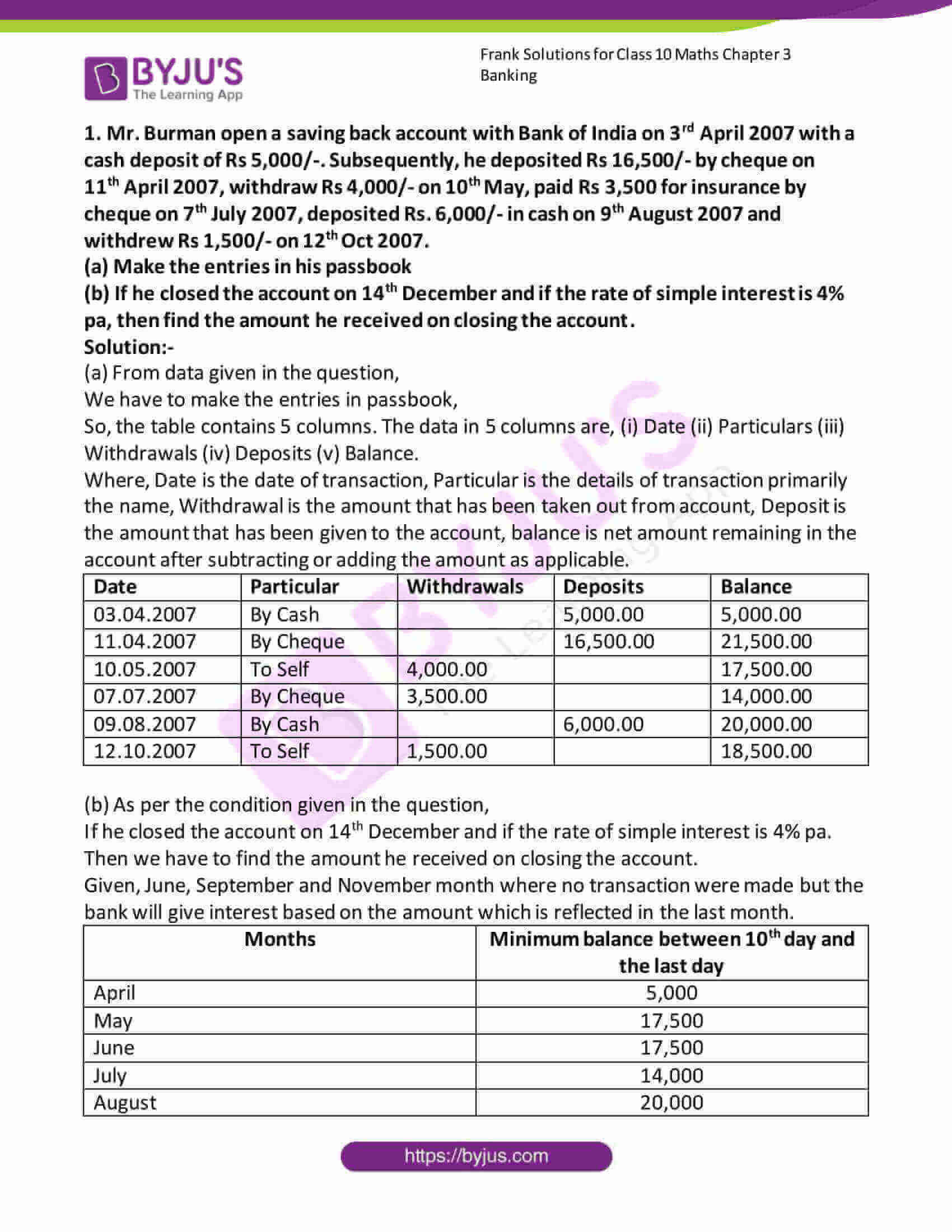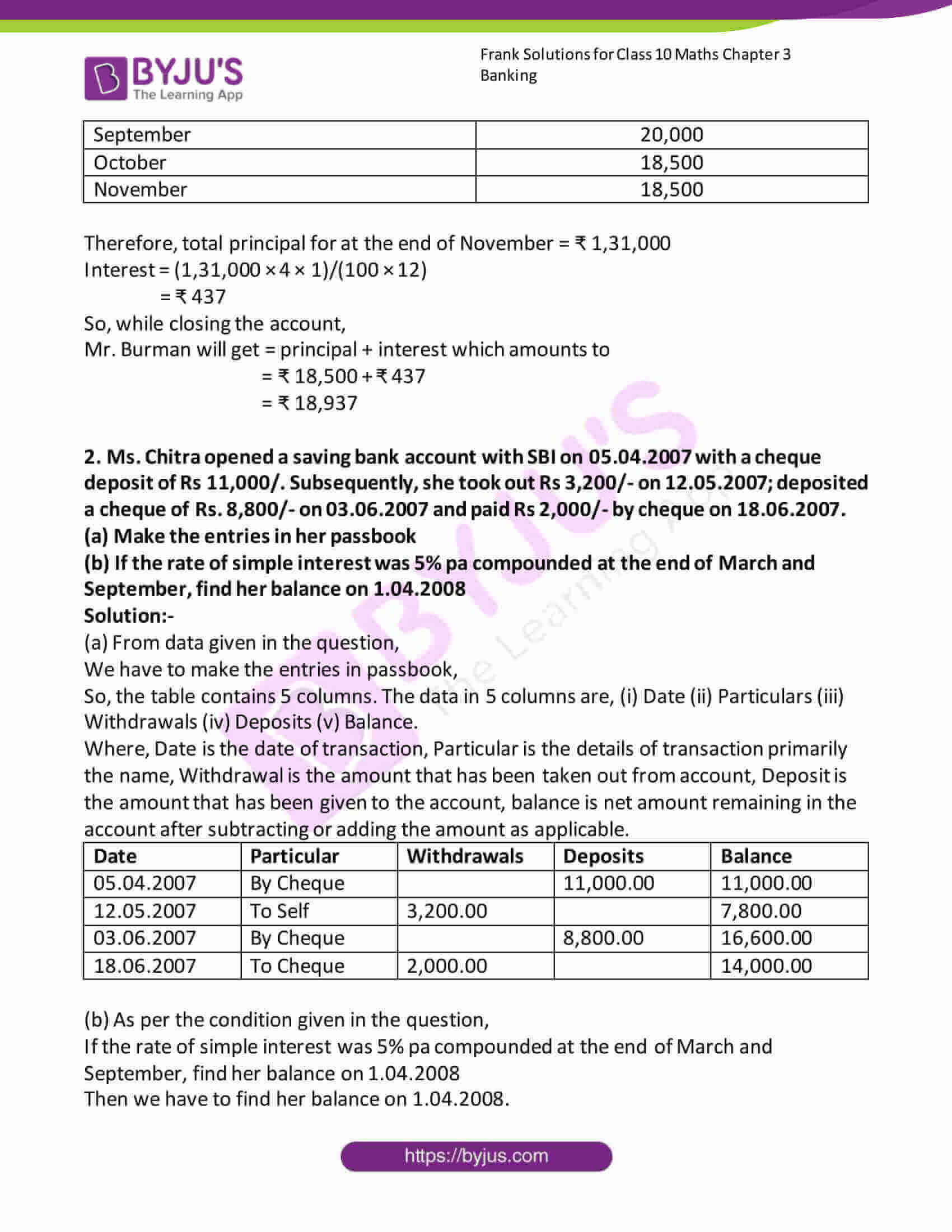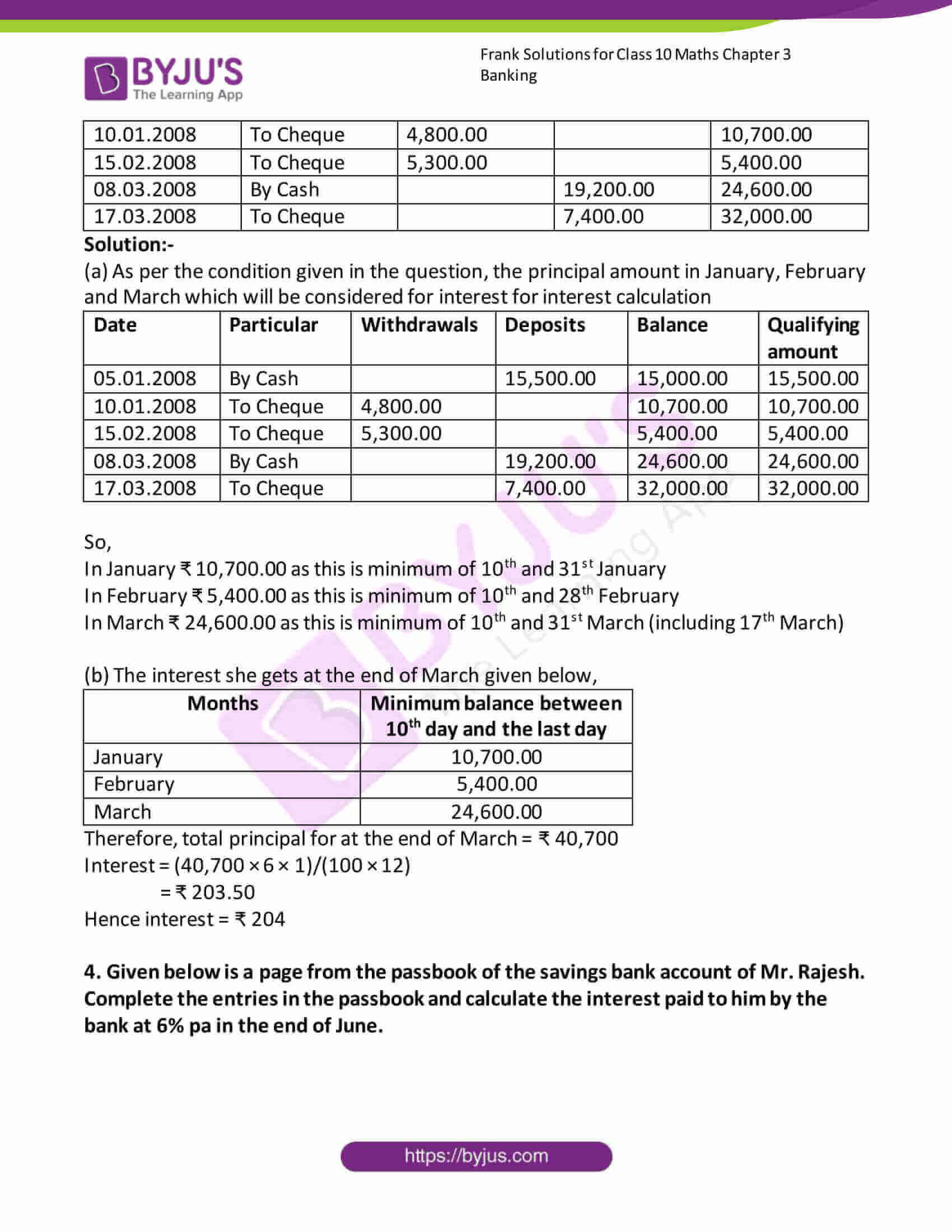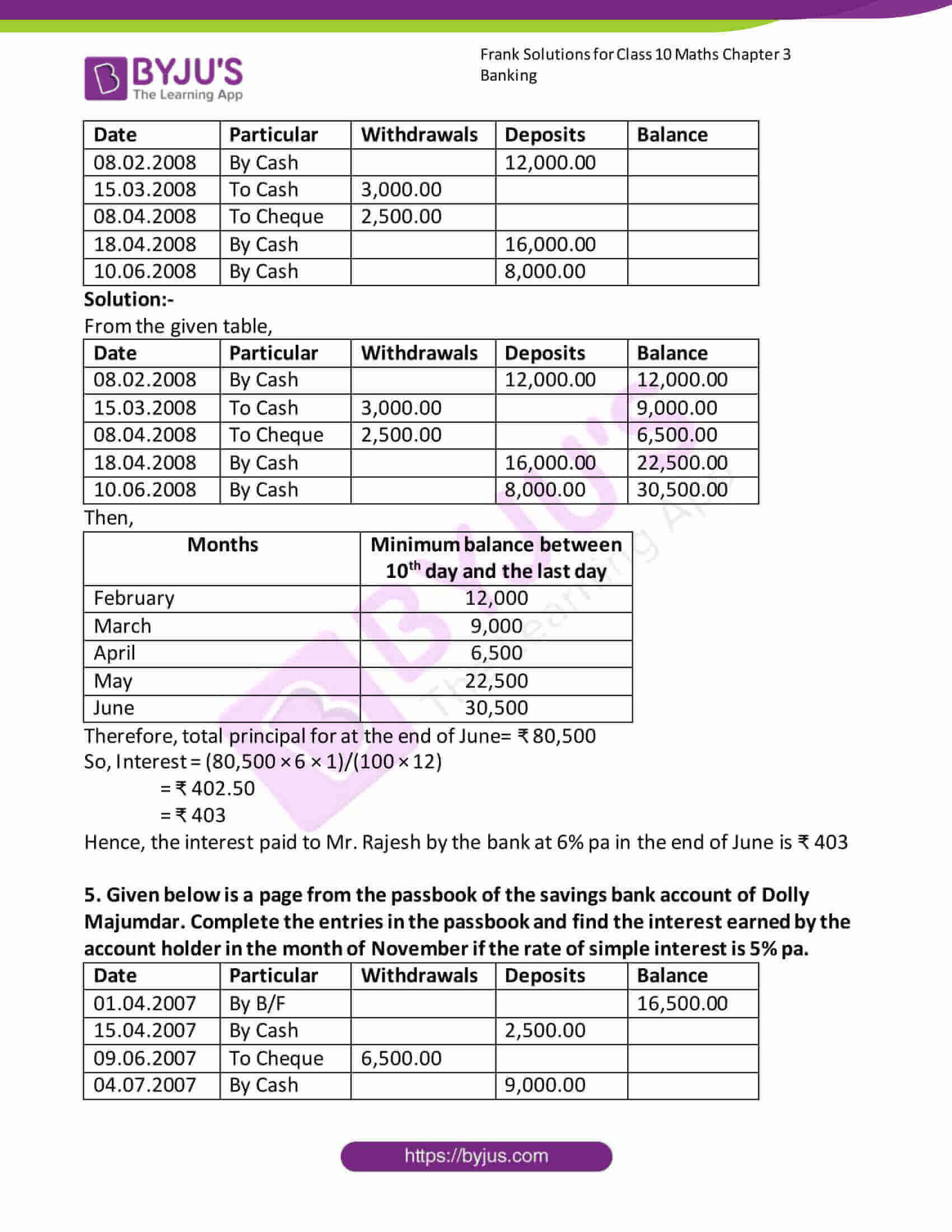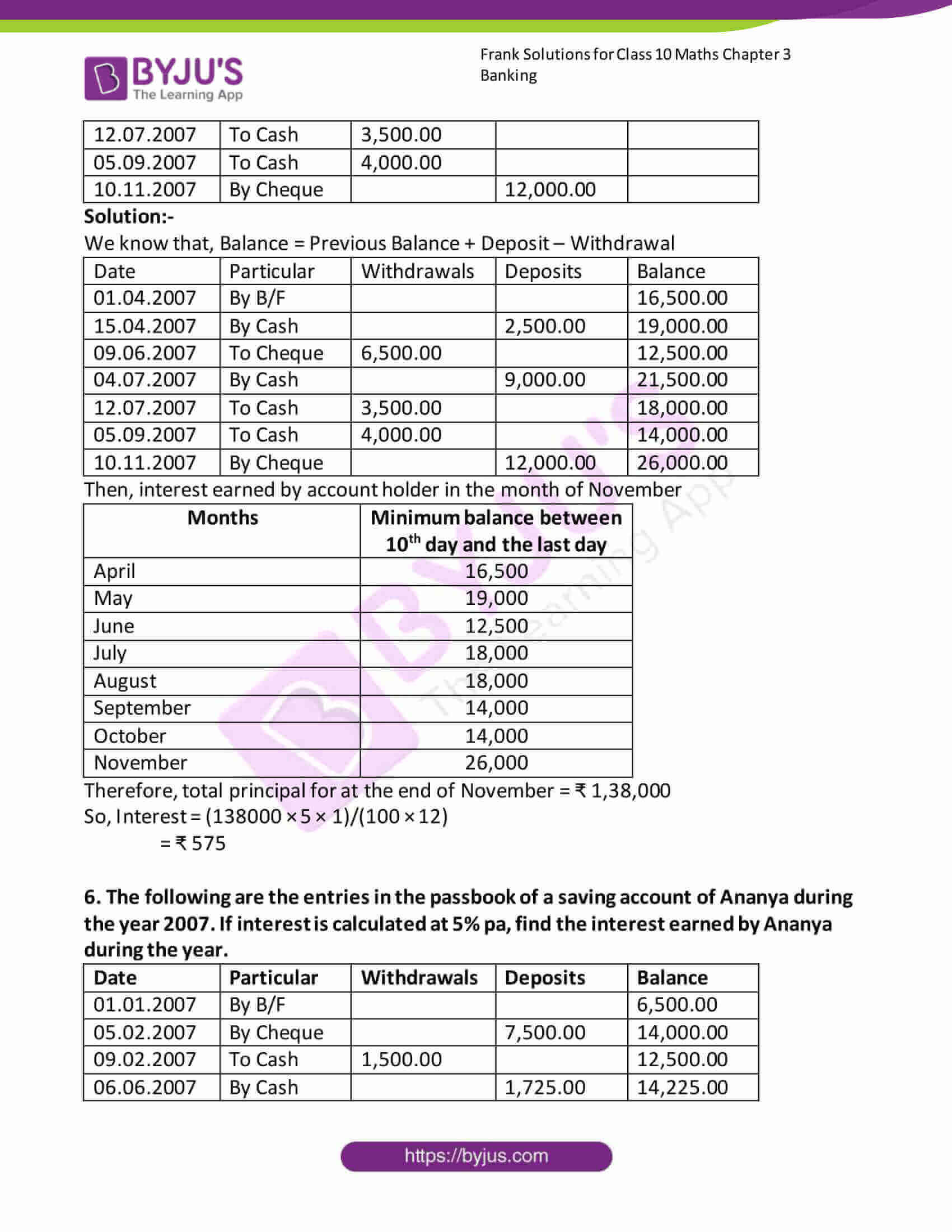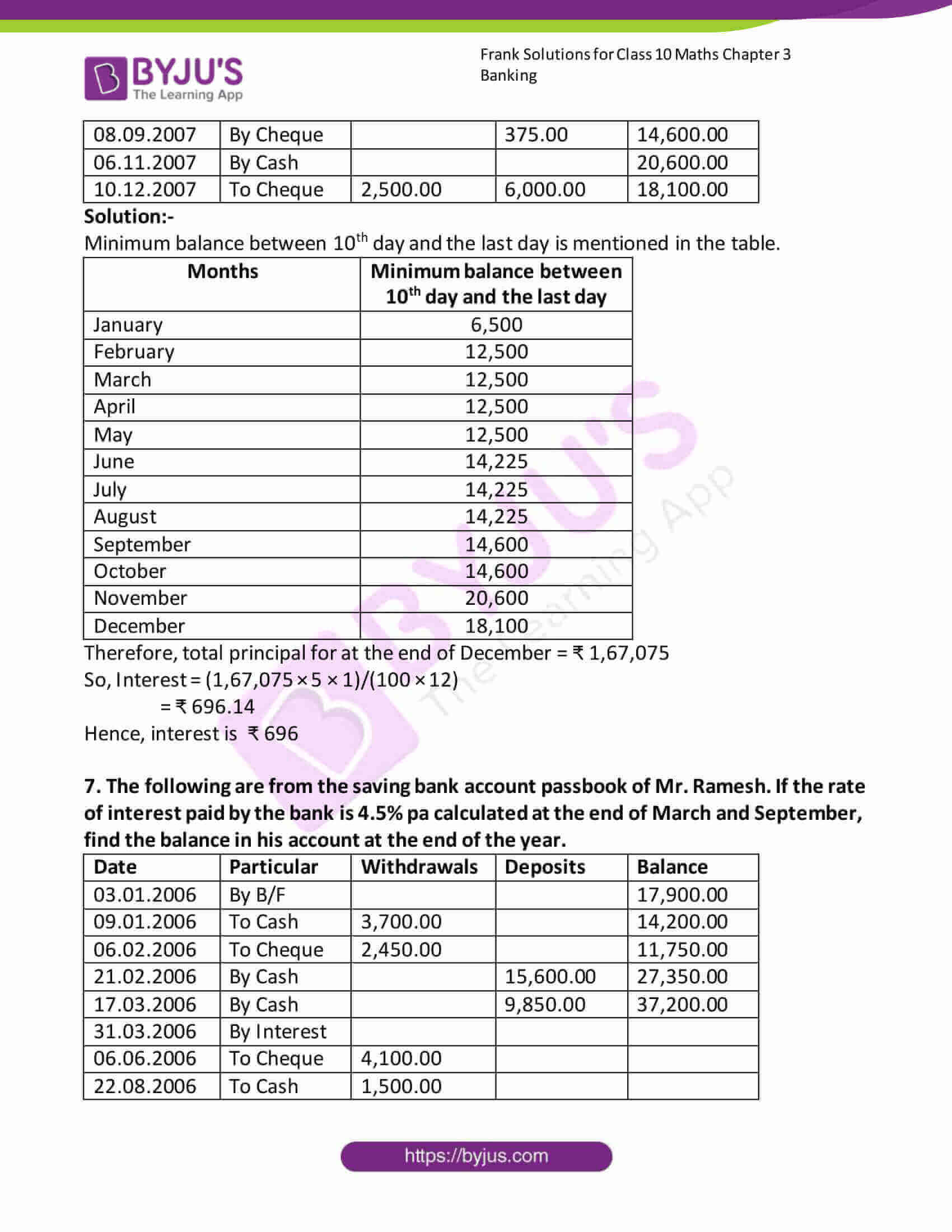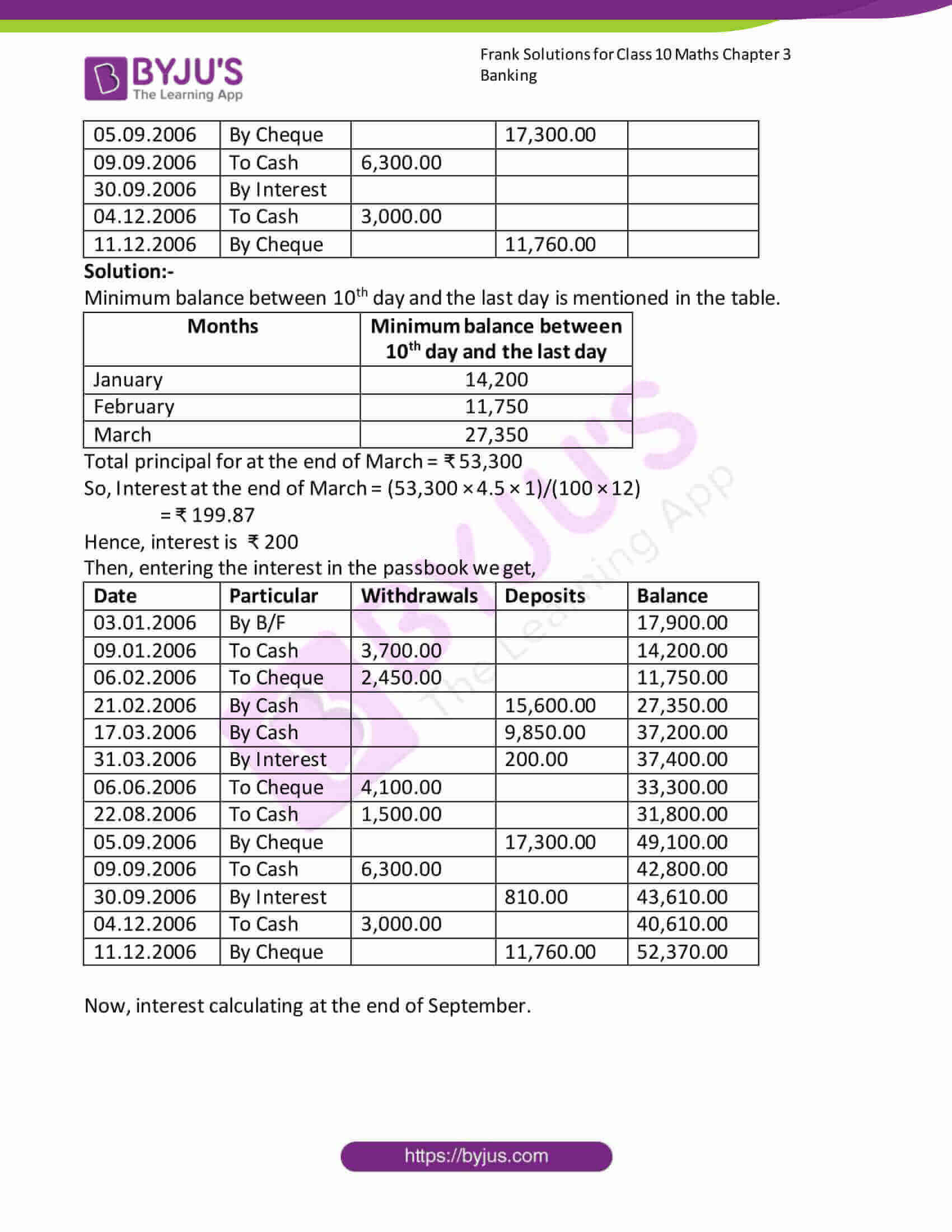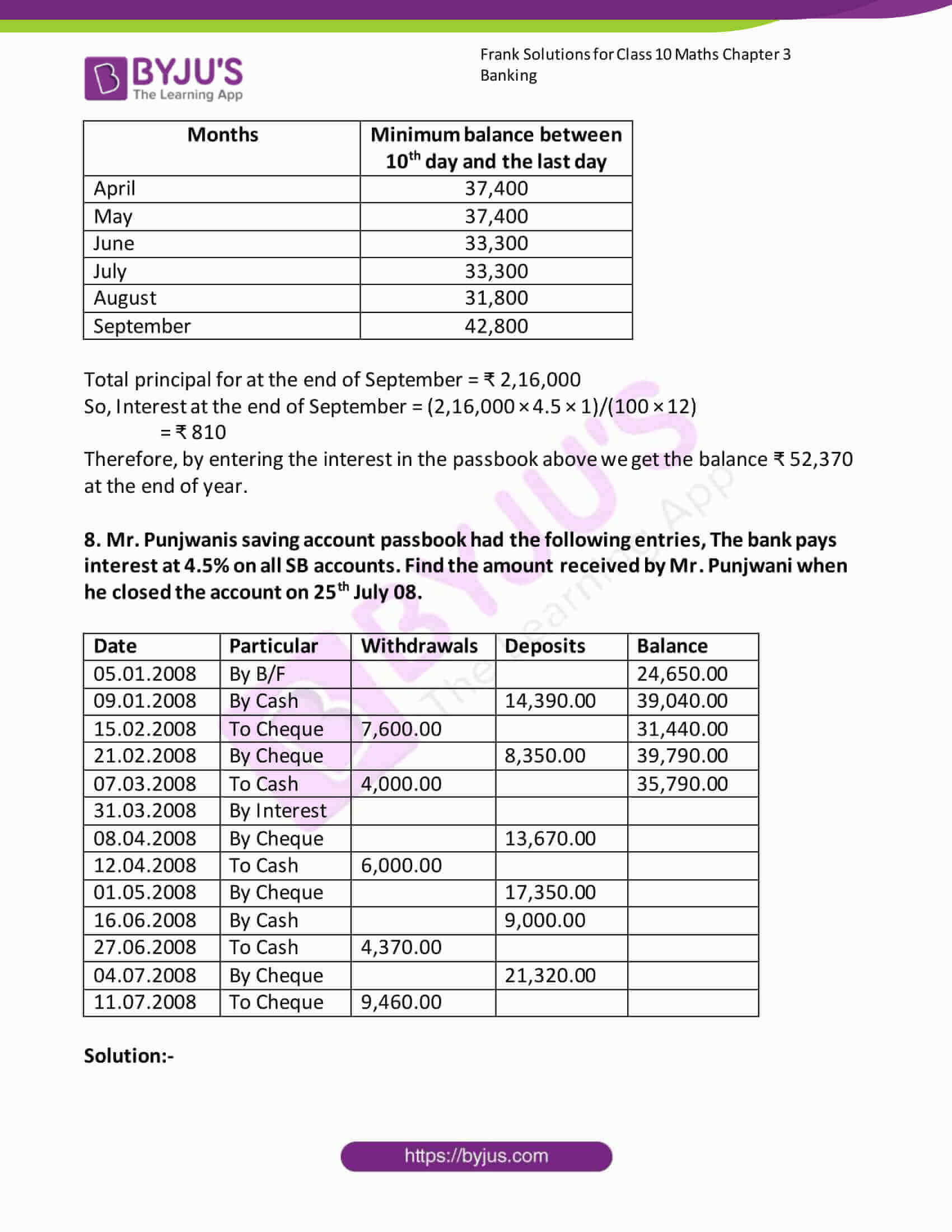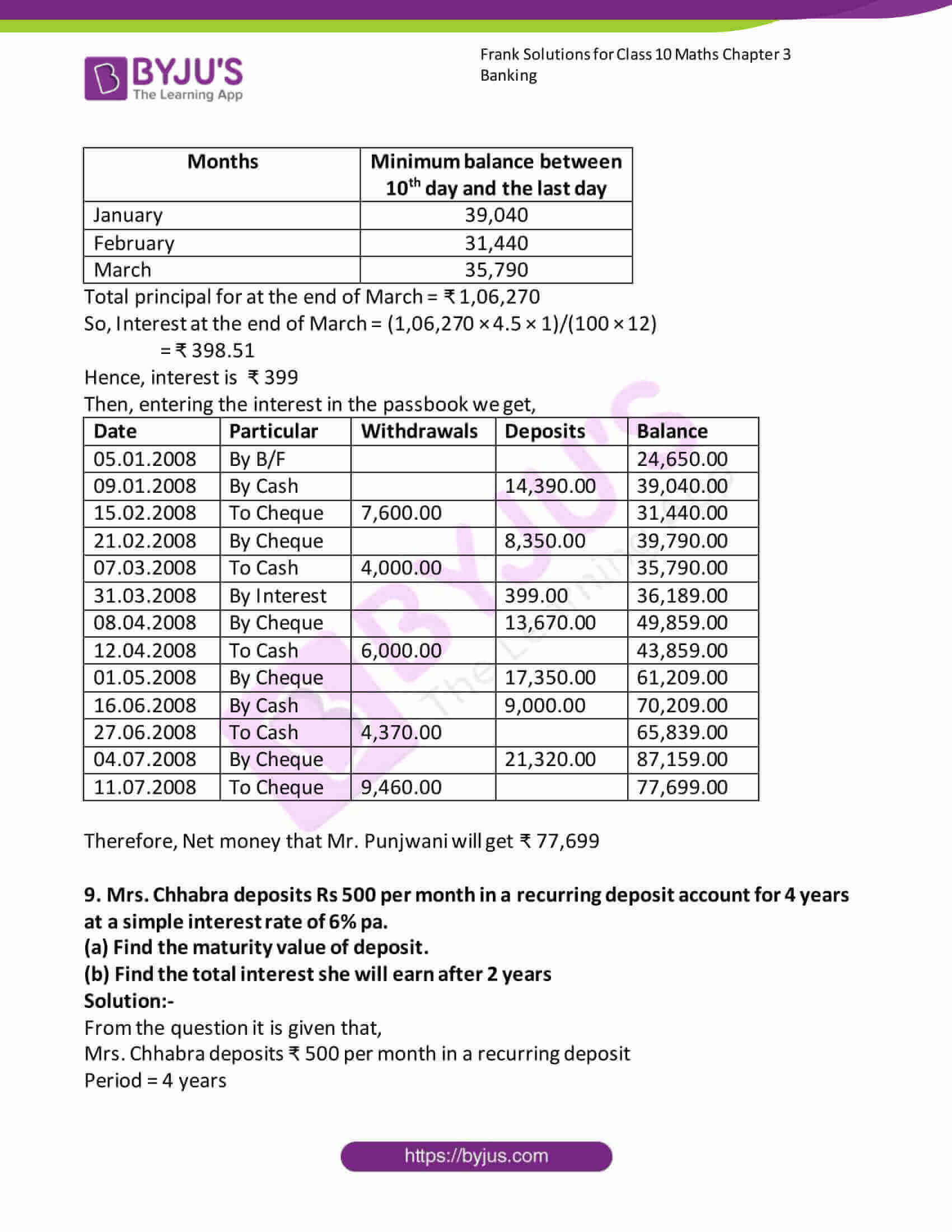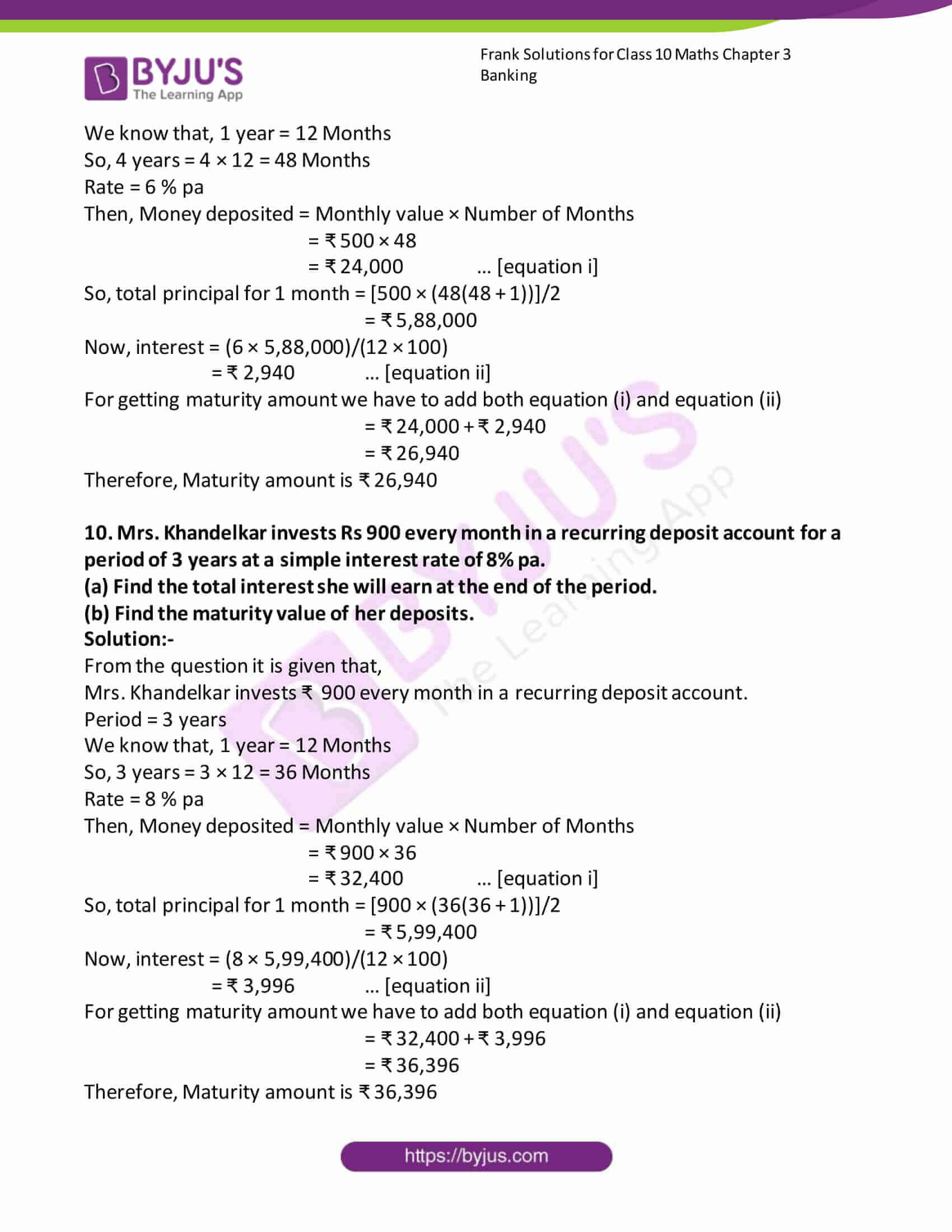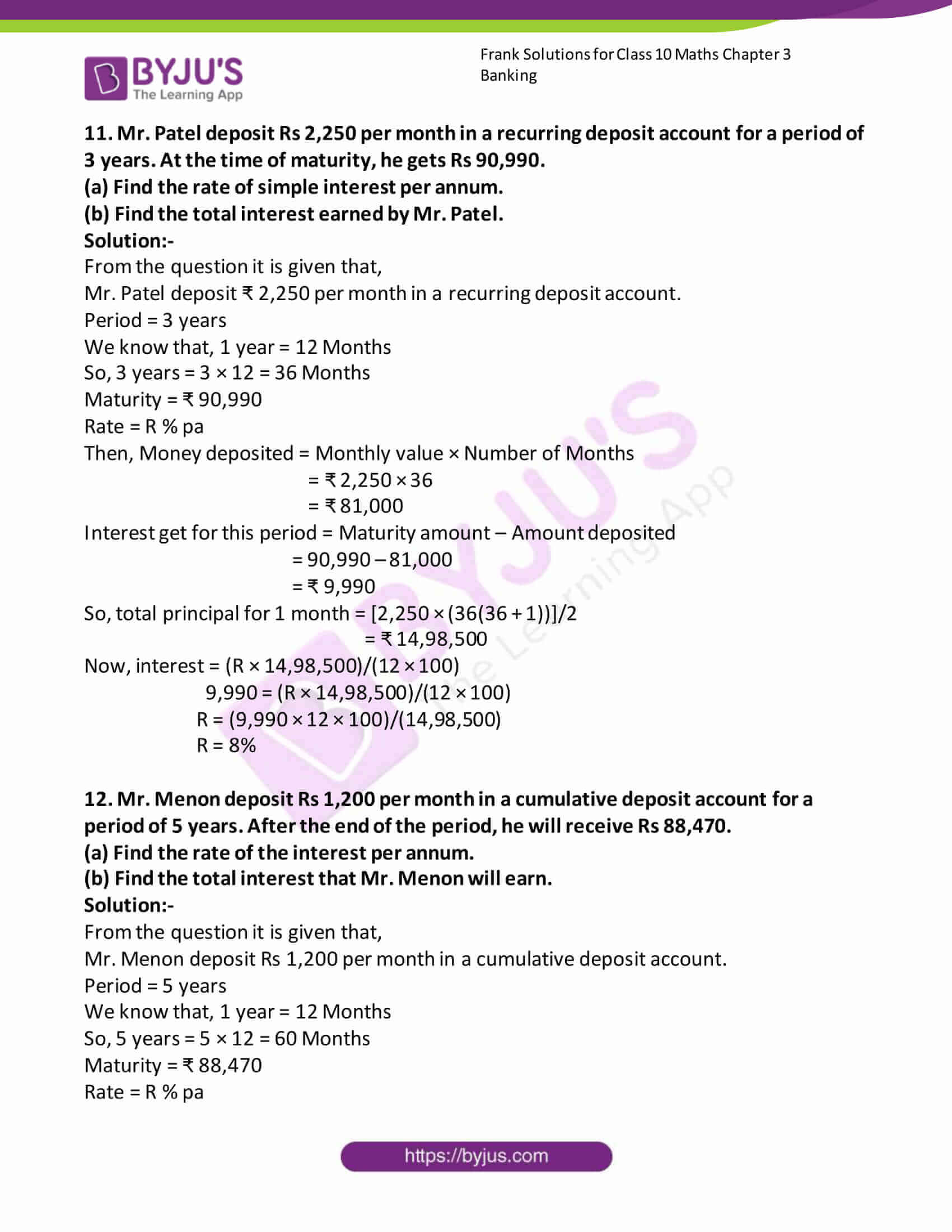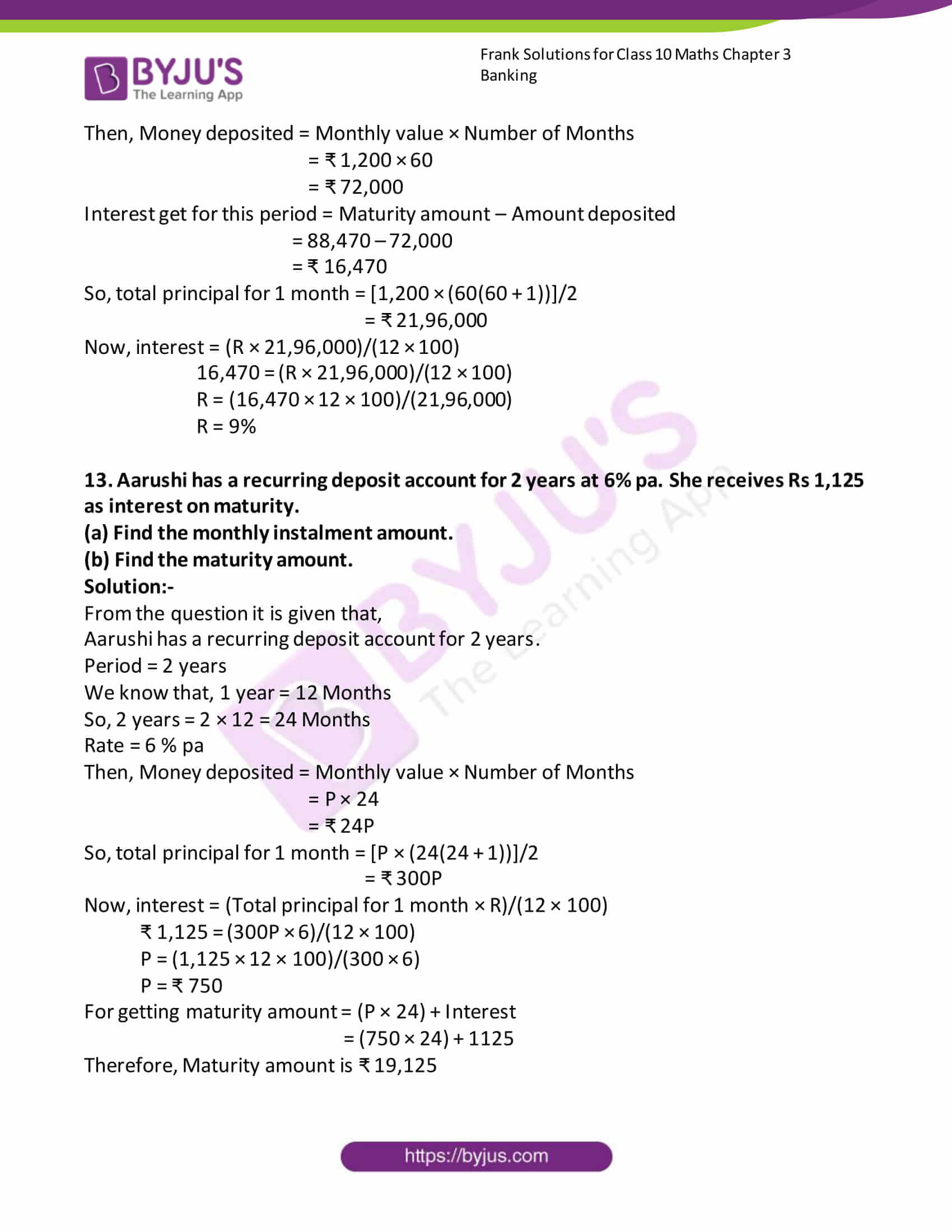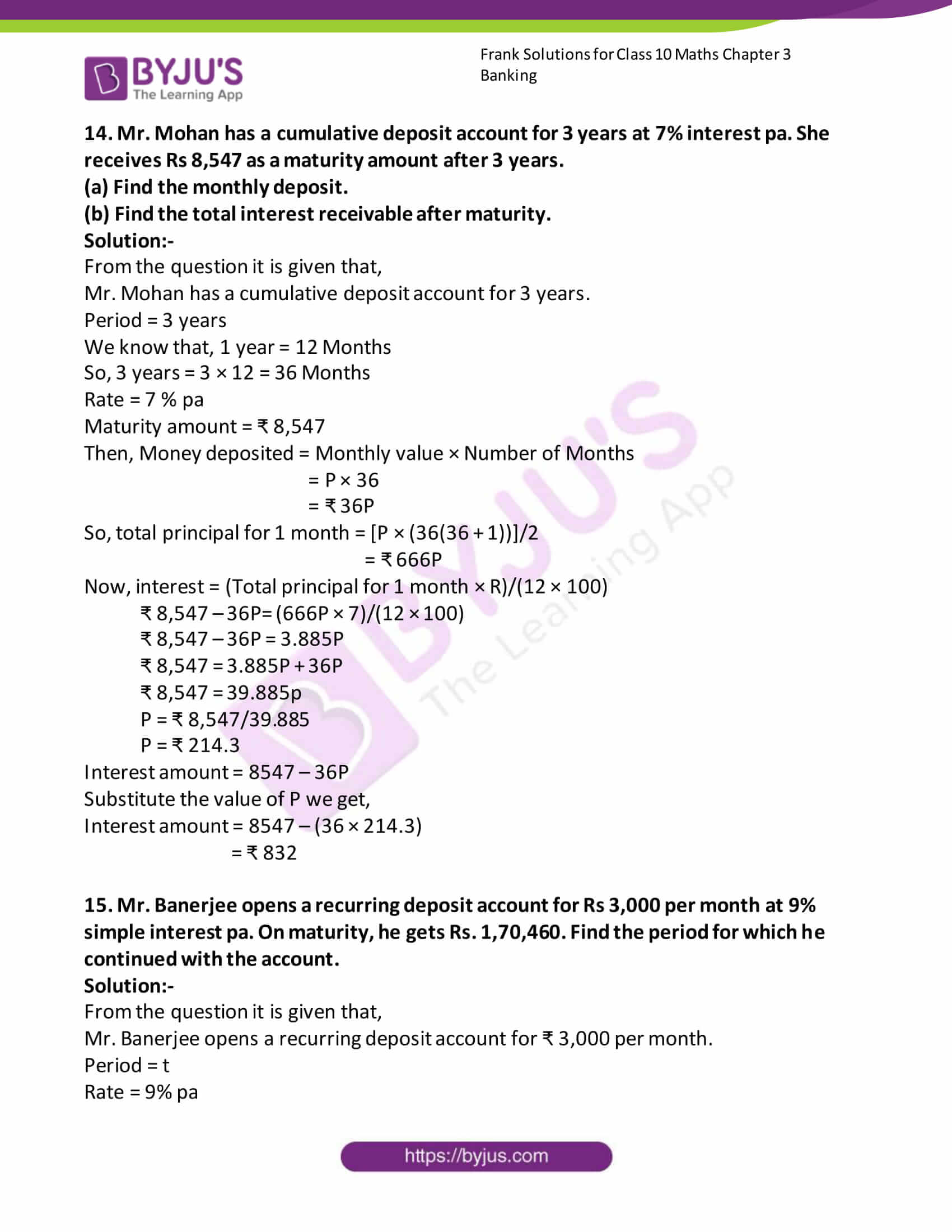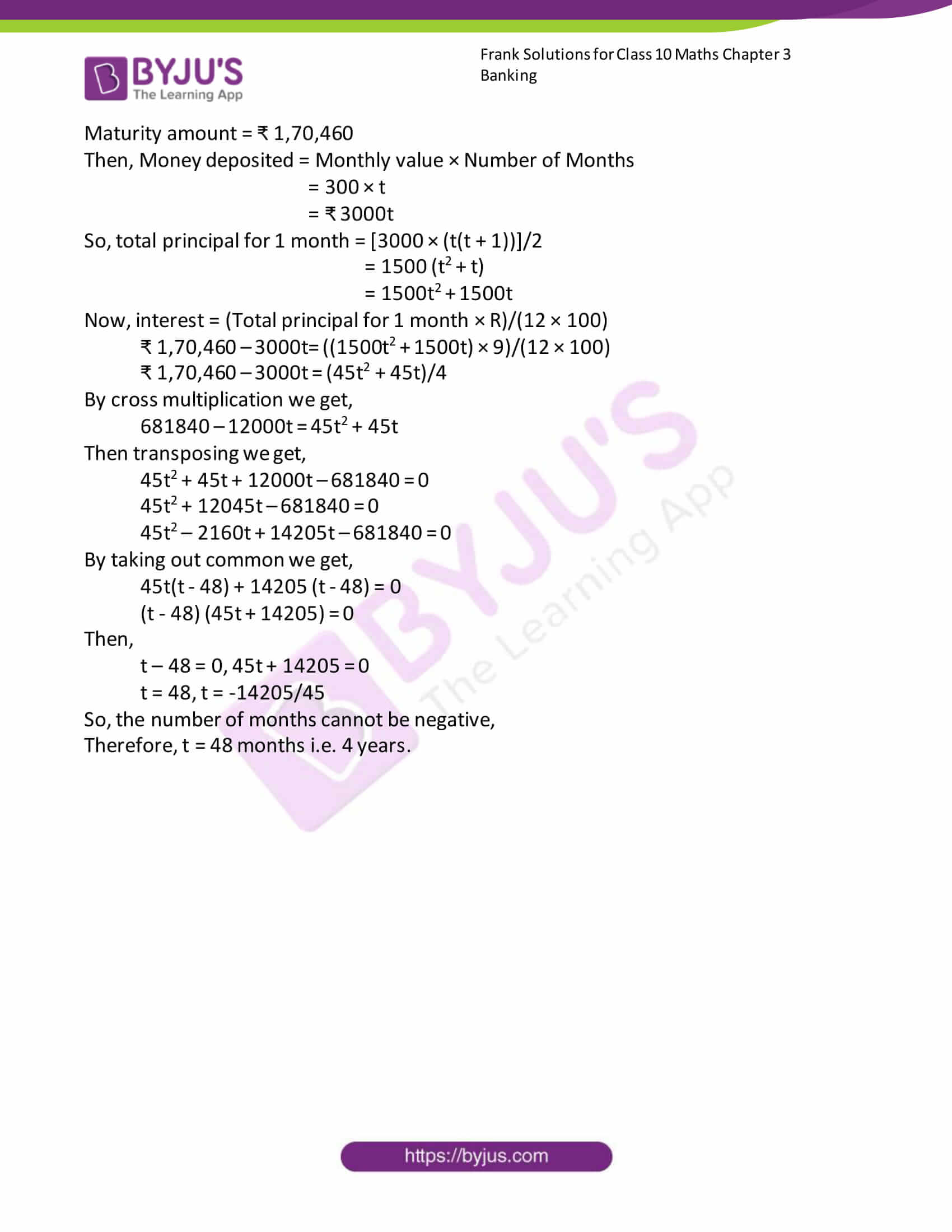### Access Answers to Frank Solutions for Class 10 Maths Chapter 3 Banking

1. Mr. Burman opened a savings bank account with Bank of India on 3rd April 2007 with a cash deposit of Rs 5,000/-. Subsequently, he deposited Rs 16,500/- by cheque on 11th April 2007, withdrew Rs 4,000/- on 10th May, paid Rs 3,500 for insurance by cheque on 7th July 2007, deposited Rs. 6,000/- in cash on 9th August 2007 and withdrew Rs 1,500/- on 12th Oct 2007.
(a) Make the entries in his passbook
(b) If he closed the account on 14th December and if the rate of simple interest is 4% pa, then find the amount he received on closing the account.

Solution:-

(a) From the data given in the question,

We have to make the entries in the passbook,

So, the table contains 5 columns. The data in 5 columns are, (i) Date (ii) Particulars (iii) Withdrawals (iv) Deposits (v) Balance.

Where date is the date of transaction, Particular is the details of the transaction, primarily the name, Withdrawal is the amount that has been taken out from the account, Deposit is the amount that has been given to the account, balance is the net amount remaining in the account after subtracting or adding the amount as applicable.

 Date Particular Withdrawals Deposits Balance 03.04.2007 By Cash 5,000.00 5,000.00 11.04.2007 By Cheque 16,500.00 21,500.00 10.05.2007 To Self 4,000.00 17,500.00 07.07.2007 By Cheque 3,500.00 14,000.00 09.08.2007 By Cash 6,000.00 20,000.00 12.10.2007 To Self 1,500.00 18,500.00

(b) As per the condition given in the question,

If he closed the account on 14th December and if the rate of simple interest is 4% pa. Then we have to find the amount he received on closing the account.

Given, June, September and November months where no transactions were made, but the bank will give interest based on the amount which is reflected in the last month.

 Months Minimum balance between the 10th day and the last day April 5,000 May 17,500 June 17,500 July 14,000 August 20,000 September 20,000 October 18,500 November 18,500

Therefore, the total principal for the end of November = ₹ 1,31,000

Interest = (1,31,000 × 4 × 1)/(100 × 12)

= ₹ 437

So, while closing the account,

Mr. Burman will get = principal + interest, which amounts to

= ₹ 18,500 + ₹ 437

= ₹ 18,937

2. Ms. Chitra opened a savings bank account with SBI on 05.04.2007 with a cheque deposit of Rs 11,000/. Subsequently, she took out Rs 3,200/- on 12.05.2007, deposited a cheque of Rs. 8,800/- on 03.06.2007 and paid Rs 2,000/- by cheque on 18.06.2007.
(a) Make the entries in her passbook
(b) If the rate of simple interest was 5% pa compounded at the end of March and September, find her balance on 1.04.2008

Solution:-

(a) From the data given in the question,

We have to make the entries in the passbook,

So, the table contains 5 columns. The data in 5 columns are, (i) Date (ii) Particulars (iii) Withdrawals (iv) Deposits (v) Balance.

Where, Date is the date of transaction, Particular is the details of the transaction, primarily the name, Withdrawal is the amount that has been taken out from the account, Deposit is the amount that has been given to the account, balance is the net amount remaining in the account after subtracting or adding the amount as applicable.

 Date Particular Withdrawals Deposits Balance 05.04.2007 By Cheque 11,000.00 11,000.00 12.05.2007 To Self 3,200.00 7,800.00 03.06.2007 By Cheque 8,800.00 16,600.00 18.06.2007 To Cheque 2,000.00 14,000.00

(b) As per the condition given in the question,

If the rate of simple interest was 5% pa compounded at the end of March and September, find her balance on 1.04.2008

Then we have to find her balance on 1.04.2008.

 Months Minimum balance between the 10th day and the last day Minimum balance in nearest multiple of 10 2007, April 11,000 11,000 May 7,800 7,800 June 14,600 14,600 July 14,600 14,600 August 14,600 14,600 September 14,600 14,600 October 14,600 + 322 = 14,922 14,920 November 14,922 14,920 December 14,922 14,920 2008, January 14,922 14,920 February 14,922 14,920 March 14,922 14,920

Therefore, the total principal for the end of September 2007,

= ₹ 11,000 + ₹ 7,800 + ₹(14,600 × 4)

= ₹ 77,200

Interest at the end of September 2007 = (77,200 × 5 × 1)/(100 × 12)

= ₹ 321.66

So, interest = ₹ 322

Then, again principal at the end of March 2008 = 14920 × 6

= ₹ 89520

Interest at the end of March 2008 = (89520 × 5 × 1)/(100 × 12)

= ₹ 373

Therefore, Account balance as on 01.04.2008 = ₹ 14920 + ₹ 373

= ₹ 15,293

3. Given below is a page from the passbook of a savings bank account that Mr. Sharma has with SBI. If the bank gives interest at 6%pa, find
(a) The principal amount in January, February and March will be considered for interest for interest calculation.
(b) The interest she gets at the end of March.

 Date Particular Withdrawals Deposits Balance 05.01.2008 By Cash 15,500.00 15,000.00 10.01.2008 To Cheque 4,800.00 10,700.00 15.02.2008 To Cheque 5,300.00 5,400.00 08.03.2008 By Cash 19,200.00 24,600.00 17.03.2008 To Cheque 7,400.00 32,000.00

Solution:-

(a) As per the condition given in the question, the principal amount in January, February and March will be considered for interest for interest calculation

 Date Particular Withdrawals Deposits Balance Qualifying amount 05.01.2008 By Cash 15,500.00 15,000.00 15,500.00 10.01.2008 To Cheque 4,800.00 10,700.00 10,700.00 15.02.2008 To Cheque 5,300.00 5,400.00 5,400.00 08.03.2008 By Cash 19,200.00 24,600.00 24,600.00 17.03.2008 To Cheque 7,400.00 32,000.00 32,000.00

So,

In January ₹ 10,700.00 as this is minimum of 10th and 31st January

In February ₹ 5,400.00 as this is minimum of 10th and 28th February

In March ₹ 24,600.00 as this is minimum of 10th and 31st March (including 17th March)

(b) The interest she gets at the end of March is given below,

 Months Minimum balance between the 10th day and the last day January 10,700.00 February 5,400.00 March 24,600.00

Therefore, the total principal at the end of March = ₹ 40,700

Interest = (40,700 × 6 × 1)/(100 × 12)

= ₹ 203.50

Hence interest = ₹ 204

4. Given below is a page from the passbook of the savings bank account of Mr. Rajesh. Complete the entries in the passbook and calculate the interest paid to him by the bank at 6% pa at the end of June.

 Date Particular Withdrawals Deposits Balance 08.02.2008 By Cash 12,000.00 15.03.2008 To Cash 3,000.00 08.04.2008 To Cheque 2,500.00 18.04.2008 By Cash 16,000.00 10.06.2008 By Cash 8,000.00

Solution:-

From the given table,

 Date Particular Withdrawals Deposits Balance 08.02.2008 By Cash 12,000.00 12,000.00 15.03.2008 To Cash 3,000.00 9,000.00 08.04.2008 To Cheque 2,500.00 6,500.00 18.04.2008 By Cash 16,000.00 22,500.00 10.06.2008 By Cash 8,000.00 30,500.00

Then,

 Months Minimum balance between the 10th day and the last day February 12,000 March 9,000 April 6,500 May 22,500 June 30,500

Therefore, the total principal at the end of June= ₹ 80,500

So, Interest = (80,500 × 6 × 1)/(100 × 12)

= ₹ 402.50

= ₹ 403

Hence, the interest paid to Mr. Rajesh by the bank at 6% pa at the end of June is ₹ 403

5. Given below is a page from the passbook of the savings bank account of Dolly Majumdar. Complete the entries in the passbook and find the interest earned by the account holder in the month of November if the rate of simple interest is 5% pa.

 Date Particular Withdrawals Deposits Balance 01.04.2007 By B/F 16,500.00 15.04.2007 By Cash 2,500.00 09.06.2007 To Cheque 6,500.00 04.07.2007 By Cash 9,000.00 12.07.2007 To Cash 3,500.00 05.09.2007 To Cash 4,000.00 10.11.2007 By Cheque 12,000.00

Solution:-

We know that, Balance = Previous Balance + Deposit – Withdrawal

 Date Particular Withdrawals Deposits Balance 01.04.2007 By B/F 16,500.00 15.04.2007 By Cash 2,500.00 19,000.00 09.06.2007 To Cheque 6,500.00 12,500.00 04.07.2007 By Cash 9,000.00 21,500.00 12.07.2007 To Cash 3,500.00 18,000.00 05.09.2007 To Cash 4,000.00 14,000.00 10.11.2007 By Cheque 12,000.00 26,000.00

Then, interest earned by the account holder in the month of November

 Months Minimum balance between the 10th day and the last day April 16,500 May 19,000 June 12,500 July 18,000 August 18,000 September 14,000 October 14,000 November 26,000

Therefore, the total principal at the end of November = ₹ 1,38,000

So, Interest = (138000 × 5 × 1)/(100 × 12)

= ₹ 575

6. The following are the entries in the passbook of a savings account of Ananya during the year 2007. If interest is calculated at 5% pa, find the interest earned by Ananya during the year.

 Date Particular Withdrawals Deposits Balance 01.01.2007 By B/F 6,500.00 05.02.2007 By Cheque 7,500.00 14,000.00 09.02.2007 To Cash 1,500.00 12,500.00 06.06.2007 By Cash 1,725.00 14,225.00 08.09.2007 By Cheque 375.00 14,600.00 06.11.2007 By Cash 20,600.00 10.12.2007 To Cheque 2,500.00 6,000.00 18,100.00

Solution:-

The minimum balance between the 10th day and the last day is mentioned in the table.

 Months Minimum balance between the 10th day and the last day January 6,500 February 12,500 March 12,500 April 12,500 May 12,500 June 14,225 July 14,225 August 14,225 September 14,600 October 14,600 November 20,600 December 18,100

Therefore, total principal for at the end of December = ₹ 1,67,075

So, Interest = (1,67,075 × 5 × 1)/(100 × 12)

= ₹ 696.14

Hence, interest is ₹ 696

7. The following is from the savings bank account passbook of Mr. Ramesh. If the rate of interest paid by the bank is 4.5% pa calculated at the end of March and September, find the balance in his account at the end of the year.

 Date Particular Withdrawals Deposits Balance 03.01.2006 By B/F 17,900.00 09.01.2006 To Cash 3,700.00 14,200.00 06.02.2006 To Cheque 2,450.00 11,750.00 21.02.2006 By Cash 15,600.00 27,350.00 17.03.2006 By Cash 9,850.00 37,200.00 31.03.2006 By Interest 06.06.2006 To Cheque 4,100.00 22.08.2006 To Cash 1,500.00 05.09.2006 By Cheque 17,300.00 09.09.2006 To Cash 6,300.00 30.09.2006 By Interest 04.12.2006 To Cash 3,000.00 11.12.2006 By Cheque 11,760.00

Solution:-

The minimum balance between the 10th day and the last day is mentioned in the table.

 Months Minimum balance between the 10th day and the last day January 14,200 February 11,750 March 27,350

Total principal for at the end of March = ₹ 53,300

So, Interest at the end of March = (53,300 × 4.5 × 1)/(100 × 12)

= ₹ 199.87

Hence, interest is ₹ 200

Then, entering the interest in the passbook, we get,

 Date Particular Withdrawals Deposits Balance 03.01.2006 By B/F 17,900.00 09.01.2006 To Cash 3,700.00 14,200.00 06.02.2006 To Cheque 2,450.00 11,750.00 21.02.2006 By Cash 15,600.00 27,350.00 17.03.2006 By Cash 9,850.00 37,200.00 31.03.2006 By Interest 200.00 37,400.00 06.06.2006 To Cheque 4,100.00 33,300.00 22.08.2006 To Cash 1,500.00 31,800.00 05.09.2006 By Cheque 17,300.00 49,100.00 09.09.2006 To Cash 6,300.00 42,800.00 30.09.2006 By Interest 810.00 43,610.00 04.12.2006 To Cash 3,000.00 40,610.00 11.12.2006 By Cheque 11,760.00 52,370.00

Now, interest is calculated at the end of September.

 Months Minimum balance between the 10th day and the last day April 37,400 May 37,400 June 33,300 July 33,300 August 31,800 September 42,800

Total principal for at the end of September = ₹ 2,16,000

So, Interest at the end of September = (2,16,000 × 4.5 × 1)/(100 × 12)

= ₹ 810

Therefore, by entering the interest in the passbook above, we get the balance ₹ 52,370 at the end of the year.

8. Mr. Punjwanis saving account passbook had the following entries, The bank pays interest at 4.5% on all SB accounts. Find the amount received by Mr. Punjwani when he closed the account on 25th July 08.

 Date Particular Withdrawals Deposits Balance 05.01.2008 By B/F 24,650.00 09.01.2008 By Cash 14,390.00 39,040.00 15.02.2008 To Cheque 7,600.00 31,440.00 21.02.2008 By Cheque 8,350.00 39,790.00 07.03.2008 To Cash 4,000.00 35,790.00 31.03.2008 By Interest 08.04.2008 By Cheque 13,670.00 12.04.2008 To Cash 6,000.00 01.05.2008 By Cheque 17,350.00 16.06.2008 By Cash 9,000.00 27.06.2008 To Cash 4,370.00 04.07.2008 By Cheque 21,320.00 11.07.2008 To Cheque 9,460.00

Solution:-

 Months Minimum balance between the 10th day and the last day January 39,040 February 31,440 March 35,790

Total principal for at the end of March = ₹ 1,06,270

So, Interest at the end of March = (1,06,270 × 4.5 × 1)/(100 × 12)

= ₹ 398.51

Hence, interest is ₹ 399

Then, entering the interest in the passbook, we get,

 Date Particular Withdrawals Deposits Balance 05.01.2008 By B/F 24,650.00 09.01.2008 By Cash 14,390.00 39,040.00 15.02.2008 To Cheque 7,600.00 31,440.00 21.02.2008 By Cheque 8,350.00 39,790.00 07.03.2008 To Cash 4,000.00 35,790.00 31.03.2008 By Interest 399.00 36,189.00 08.04.2008 By Cheque 13,670.00 49,859.00 12.04.2008 To Cash 6,000.00 43,859.00 01.05.2008 By Cheque 17,350.00 61,209.00 16.06.2008 By Cash 9,000.00 70,209.00 27.06.2008 To Cash 4,370.00 65,839.00 04.07.2008 By Cheque 21,320.00 87,159.00 11.07.2008 To Cheque 9,460.00 77,699.00

Therefore, the net money that Mr. Punjwani will get ₹ 77,699

9. Mrs. Chhabra deposits Rs 500 per month in a recurring deposit account for 4 years at a simple interest rate of 6% pa.
(a) Find the maturity value of the deposit.
(b) Find the total interest she will earn after 2 years

Solution:-

From the question, it is given that,

Mrs. Chhabra deposits ₹ 500 per month in a recurring deposit

Period = 4 years

We know that, 1 year = 12 Months

So, 4 years = 4 × 12 = 48 Months

Rate = 6 % pa

Then, Money deposited = Monthly value × Number of Months

= ₹ 500 × 48

= ₹ 24,000 … [equation i]

So, total principal for 1 month = [500 × (48(48 + 1))]/2

= ₹ 5,88,000

Now, interest = (6 × 5,88,000)/(12 × 100)

= ₹ 2,940 … [equation ii]

To get the maturity amount, we have to add both equation (i) and equation (ii)

= ₹ 24,000 + ₹ 2,940

= ₹ 26,940

Therefore, the maturity amount is ₹ 26,940

10. Mrs. Khandelkar invests Rs 900 every month in a recurring deposit account for a period of 3 years at a simple interest rate of 8% pa.
(a) Find the total interest she will earn at the end of the period.
(b) Find the maturity value of her deposits.

Solution:-

From the question, it is given that,

Mrs. Khandelkar invests ₹ 900 every month in a recurring deposit account.

Period = 3 years

We know that, 1 year = 12 Months

So, 3 years = 3 × 12 = 36 Months

Rate = 8 % pa

Then, Money deposited = Monthly value × Number of Months

= ₹ 900 × 36

= ₹ 32,400 … [equation i]

So, total principal for 1 month = [900 × (36(36 + 1))]/2

= ₹ 5,99,400

Now, interest = (8 × 5,99,400)/(12 × 100)

= ₹ 3,996 … [equation ii]

To get the maturity amount, we have to add both equation (i) and equation (ii)

= ₹ 32,400 + ₹ 3,996

= ₹ 36,396

Therefore, the Maturity amount is ₹ 36,396

11. Mr. Patel deposits Rs 2,250 per month in a recurring deposit account for a period of 3 years. At the time of maturity, he gets Rs 90,990.
(a) Find the rate of simple interest per annum.
(b) Find the total interest earned by Mr. Patel.

Solution:-

From the question it is given that,

Mr. Patel deposits ₹ 2,250 per month in a recurring deposit account.

Period = 3 years

We know that, 1 year = 12 Months

So, 3 years = 3 × 12 = 36 Months

Maturity = ₹ 90,990

Rate = R % pa

Then, Money deposited = Monthly value × Number of Months

= ₹ 2,250 × 36

= ₹ 81,000

Interest get for this period = Maturity amount – Amount deposited

= 90,990 – 81,000

= ₹ 9,990

So, total principal for 1 month = [2,250 × (36(36 + 1))]/2

= ₹ 14,98,500

Now, interest = (R × 14,98,500)/(12 × 100)

9,990 = (R × 14,98,500)/(12 × 100)

R = (9,990 × 12 × 100)/(14,98,500)

R = 8%

12. Mr. Menon deposits Rs 1,200 per month in a cumulative deposit account for a period of 5 years. After the end of the period, he will receive Rs 88,470.

(a) Find the rate of the interest per annum.

(b) Find the total interest that Mr. Menon will earn.

Solution:-

From the question, it is given that,

Mr. Menon deposits Rs 1,200 per month in a cumulative deposit account.

Period = 5 years

We know that, 1 year = 12 Months

So, 5 years = 5 × 12 = 60 Months

Maturity = ₹ 88,470

Rate = R % pa

Then, Money deposited = Monthly value × Number of Months

= ₹ 1,200 × 60

= ₹ 72,000

Interest get for this period = Maturity amount – Amount deposited

= 88,470 – 72,000

= ₹ 16,470

So, total principal for 1 month = [1,200 × (60(60 + 1))]/2

= ₹ 21,96,000

Now, interest = (R × 21,96,000)/(12 × 100)

16,470 = (R × 21,96,000)/(12 × 100)

R = (16,470 × 12 × 100)/(21,96,000)

R = 9%

13. Aarushi has a recurring deposit account for 2 years at 6% pa. She receives Rs 1,125 as interest on maturity.
(a) Find the monthly instalment amount.
(b) Find the maturity amount.

Solution:-

From the question, it is given that,

Aarushi has a recurring deposit account for 2 years.

Period = 2 years

We know that, 1 year = 12 Months

So, 2 years = 2 × 12 = 24 Months

Rate = 6 % pa

Then, Money deposited = Monthly value × Number of Months

= P × 24

= ₹ 24P

So, total principal for 1 month = [P × (24(24 + 1))]/2

= ₹ 300P

Now, interest = (Total principal for 1 month × R)/(12 × 100)

₹ 1,125 = (300P × 6)/(12 × 100)

P = (1,125 × 12 × 100)/(300 × 6)

P = ₹ 750

For getting maturity amount = (P × 24) + Interest

= (750 × 24) + 1125

Therefore, the maturity amount is ₹ 19,125

14. Mr. Mohan has a cumulative deposit account for 3 years at 7% interest pa. She receives Rs 8,547 as a maturity amount after 3 years.
(a) Find the monthly deposit.
(b) Find the total interest receivable after maturity.

Solution:-

From the question, it is given that,

Mr. Mohan has a cumulative deposit account for 3 years.

Period = 3 years

We know that, 1 year = 12 Months

So, 3 years = 3 × 12 = 36 Months

Rate = 7 % pa

Maturity amount = ₹ 8,547

Then, Money deposited = Monthly value × Number of Months

= P × 36

= ₹ 36P

So, total principal for 1 month = [P × (36(36 + 1))]/2

= ₹ 666P

Now, interest = (Total principal for 1 month × R)/(12 × 100)

₹ 8,547 – 36P= (666P × 7)/(12 × 100)

₹ 8,547 – 36P = 3.885P

₹ 8,547 = 3.885P + 36P

₹ 8,547 = 39.885p

P = ₹ 8,547/39.885

P = ₹ 214.3

Interest amount = 8547 – 36P

Substitute the value of P we get,

Interest amount = 8547 – (36 × 214.3)

= ₹ 832

15. Mr. Banerjee opens a recurring deposit account for Rs 3,000 per month at 9% simple interest pa. On maturity, he gets Rs. 1,70,460. Find the period for which he continued with the account.

Solution:-

From the question, it is given that,

Mr. Banerjee opens a recurring deposit account for ₹ 3,000 per month.

Period = t

Rate = 9% pa

Maturity amount = ₹ 1,70,460

Then, Money deposited = Monthly value × Number of Months

= 300 × t

= ₹ 3000t

So, total principal for 1 month = [3000 × (t(t + 1))]/2

= 1500 (t2 + t)

= 1500t2 + 1500t

Now, interest = (Total principal for 1 month × R)/(12 × 100)

₹ 1,70,460 – 3000t= ((1500t2 + 1500t) × 9)/(12 × 100)

₹ 1,70,460 – 3000t = (45t2 + 45t)/4

By cross multiplication, we get,

681840 – 12000t = 45t2 + 45t

Then transposing, we get,

45t2 + 45t + 12000t – 681840 = 0

45t2 + 12045t – 681840 = 0

45t2 – 2160t + 14205t – 681840 = 0

By taking out common, we get,

45t(t – 48) + 14205 (t – 48) = 0

(t – 48) (45t + 14205) = 0

Then,

t – 48 = 0, 45t + 14205 = 0

t = 48, t = -14205/45

So, the number of months cannot be negative,

Therefore, t = 48 months, i.e. 4 years.